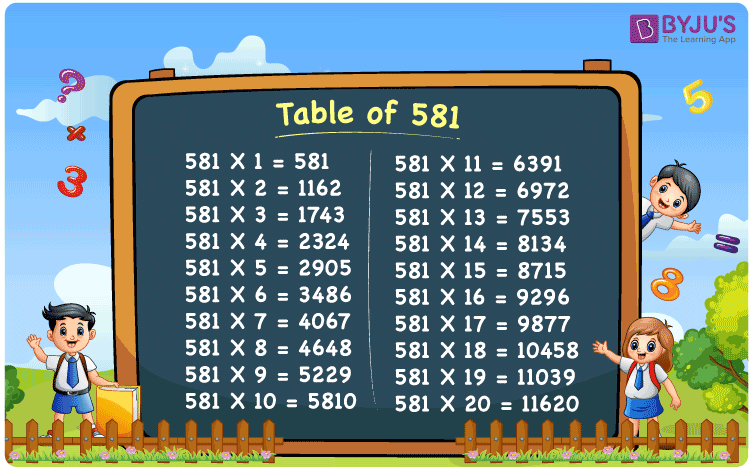Checkout JEE MAINS 2022 Question Paper Analysis : Checkout JEE MAINS 2022 Question Paper Analysis :

# Table of 581

The Table of 581 is presented here to help the students quickly check the values of the 581 times table up to 20. The multiplication table of 581 is given below in a tabular format, and a PDF is also provided to download. Students can download the PDF and use the image as a flashcard, which will help them memorise the tables.

## Table of 581 Chart

The table chart of 581 comes in handy for a quick check with the values of multiples of 581. Apart from that, the image can be used as flashcards while doing mental maths and learning the table of 581.## What is the 581 Times Table?

The 581 times table is the multiplication of 581 with the natural numbers. The 581 times table can be obtained both by multiplication and by the addition of 581 repeatedly. The below chart shows the multiplication table of 581, in both multiplication and addition form.

581 × 1 = 581

581

581 × 2 = 1162

581 + 581 = 1162

581 × 3 = 1743

581 + 581 + 581 = 1743

581 × 4 = 2324

581 + 581 + 581 + 581 = 2324

581 × 5 = 2905

581 + 581 + 581 + 581 + 581 = 2905

581 × 6 = 3486

581 + 581 + 581 + 581 + 581 + 581 = 3486

581 × 7 = 4067

581 + 581 + 581 + 581 + 581 + 581 + 581 = 4067

581 × 8 = 4648

581 + 581 + 581 + 581 + 581 + 581 + 581 + 581 = 4648

581 × 9 = 5229

581 + 581 + 581 + 581 + 581 + 581 + 581 + 581 + 581 = 5229

581 × 10 = 5810

581 + 581 + 581 + 581 + 581 + 581 + 581 + 581 + 581 + 581 = 5810

## Multiplication Table of 581

Given below are the first 20 multiples of 581 that are obtained by the multiplication of the first 20 natural numbers with 581.

 581 × 1 = 581 581 × 2 = 1162 581 × 3 = 1743 581 × 4 = 2324 581 × 5 = 2905 581 × 6 = 3486 581 × 7 = 4067 581 × 8 = 4648 581 × 9 = 5229 581 × 10 = 5810 581 × 11 = 6391 581 × 12 = 6972 581 × 13 = 7553 581 × 14 = 8134 581 × 15 = 8715 581 × 16 = 9296 581 × 17 = 9877 581 × 18 = 10458 581 × 19 = 11039 581 × 20 = 11620

## Solved Examples on the Table of 581

Example 1:

What is five more than 17 times of 581?

Solution:

17 times 581 = 581 × 17  = 9877

Five more than 9877 = 9877 + 5 = 9882.

## Frequently Asked Questions on the Table of 581

### What is the 581 times table?

The 581 times table is the multiplication table of 581 with given natural numbers. For example, 581 × 1 = 581, 581 × 2 = 1162, 581 × 3 = 1743 and so on.

### What times table has 581?

581 comes in the times table of 1, 7, 83 and 581.

### Does 581 come in 7 times table?

Yes, 581 comes in the times table of 7, 83 × 7 = 581.